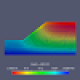Many people develop their OpenSees elements and materials in MATLAB, then port to C++. To support this transition, OpenSees implements easy matrix-vector algebra by overloading the `+`, `-`, `*`, and `^` operators for the Matrix and Vector classes. The overloaded operators are self-explanatory, except for `^`, which is “transpose times” or inner product.

``````C = A^B; // A'*B in MATLAB
C = A^B*A; // A'*B*A in MATLAB``````

But if you care about efficiency, you should dispense with the overloaded operators after confirming your model passes its patch tests, converges quadratically, produces no memory leaks, etc.

Every time they are called, the overloaded operators produce a temporary Matrix or Vector object, which is costly when dealing with operations at the low levels of the OpenSees hierarchy–operations that could be called millions of times in a single response history analysis.

To avoid the computational overhead of the overloaded operators, several daxpy-like functions are available in the Matrix and Vector classes. For example, addMatrixTripleProduct() does exactly what its name implies.

``````// C = a*C + b*A^B*A

The first scalar, `a`, is the thisFactor applied to the left-hand side, while the second scalar, `b`, at the end of the argument list, is the otherFactor applied to the right-hand side. Any scalars can be used, but most often you will use `a=0` or `1` with `b=1`. For these common cases, the daxpy-like functions react accordingly to bypass unnecessary multiplies by zero or one.

``````// a = 0, b = 1 --> C = A^B*A

// a = 1, b = 1 --> = C = C + A^B*A

// a = 0.5, b = -2 --> C = 0.5*C - 2*A^B*A

To see these functions in action, consider the mixed beam-column element coded by Prof. Mark Denavit. The following line of code appears in MixedBeamColumn3d::update().

``K_temp = ( Kg + G2 + G2T - H22 ) + GMHT * Hinv * GMH;``

There’s a lot going on in this single line of code–four matrix additions and two matrix multiplications using overloaded operators.

Noting that `G2T` and `GMHT` are the transposes of `G2` and `GMH`, respectively, we can turn that line of MATLAB-like code into the following sequence of function calls.

``````K_temp = Kg; // Kg
K_temp.addMatrix(1.0, G2, 1.0); // + G2
K_temp.addMatrixTranspose(1.0, G2, 1.0); // + G2T
K_temp.addMatrix(1.0, H22, -1.0); // - H22
K_temp.addMatrixTripleProduct(1.0, GMH, Hinv, 1.0); // + GMHT*Hinv*GMH``````

Yes, it’s more work to write out these lines of code, but we avoid a lot of temporary Matrix objects and the code will run faster. We also don’t need to store `G2T` and `GMHT`.

To assess the speedup, I wrote a short main() function to time two implementations of the same matrix operation. Loop 1 uses overloaded operators while Loop 2 uses the addMatrixTripleProduct() function to calculate and assign a matrix triple product.

``````Matrix A(N,N);
Matrix B(N,N);
Matrix C(N,N);

// Fill A and B with random values

const int n = 1000000;

// Loop 1
for (int i = 0; i < n; i++) {
C = B^A*B;
}

// Loop 2
for (int i = 0; i < n; i++) {
}``````

A million trials smooth out the variance in execution time of individual operations. Although not shown in the code above, I used the best answer from this Stack Overflow question to time the loops.

The ratio of execution time for Loop 1 relative to Loop 2 is shown below. For small matrices (N<5), the overloaded operators take much longer to execute relative to the daxpy-like function. As the matrix size increases, the ratio hovers just above one because the raw math takes longer relative to the memory management.

This comparison represents what would be but one operation within the larger maelstrom of operations that constitute an OpenSees analysis. But, the minor victories add up.

Un-MATLAB your OpenSees C++ code, i.e., change from overloaded operators to the daxpy-like functions, and you’ll see noticeable reductions in run time. Prof. Denavit has some work to do–but he’s not the only one.

Further speedup can be gained by unrolling algebraic operations to exploit matrix sparsity and/or symmetry. I’ll address that in another post.

## 6 thoughts on “Un-MATLAB Your OpenSees”

1.Sukumar Baishya says:

Thanks Prof Scott for this post. Fortunately, it’s a great and timely guidance for me on the job of implementing a new element ( different from FEM, but with some FEM like features). I was thinking about discussing the matter with you over email, these days..
Best regards.

Liked by 1 person

2.Mark Denavit says:

Hey, I like MATLAB!

Like

1.Mark Denavit says:

But seriously, thanks. I knew about these functions when coding the element, but what they did was a total mystery to me.

Liked by 1 person

2.Michael H. Scott says:

Me too. I wasn’t disparaging MATLAB!

Like

This site uses Akismet to reduce spam. Learn how your comment data is processed.